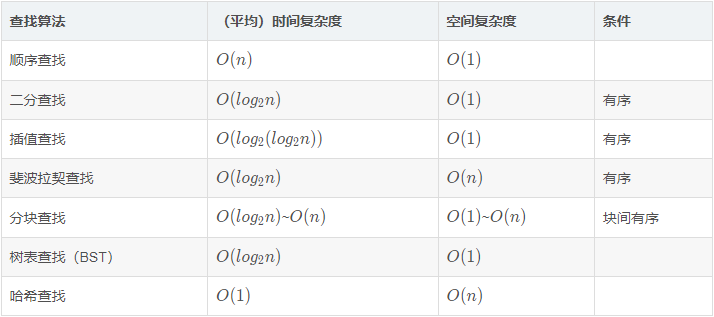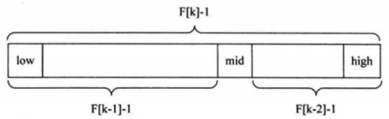## 1、概述

### 1.1 查找算法分类

1. 静态查找和动态查找

注：静态或者动态都是针对查找表而言的。动态表指查找表中有删除和插入操作的表

2. 无序查找和有序查找

• 无序查找：被查找数列有序无序均可
• 有序查找：被查找数列必须为有序数列

### 1.2 复杂度性质汇总

• P i：查找表中第i个数据元素的概率。
• C i：找到第i个数据元素时已经比较过的次数## 2、顺序（线性）查找

public static int search(int[] arr, int val) {
int length = arr.length;
for (int i = 0; i < length; i++) {
if (val == arr[i]) {
return arr[i];
}
}
return -1;
}

## 3、二分（折半）查找

1. 首先确定该数组的中间的下标，mid = (left + right) / 2;
2. 然后让需要查找的数 findValarr[mid] 比较
• 如果 findVal < arr[mid]，说明需要查找的数在 mid 左边，因此需要继续递归向左查找
• 如果 findVal > arr[mid]，说明需要查找的数在 mid 右边，因此需要继续递归向右查找
• 如果 findVal = arr[mid]，说明需要查找的数位置就是 mid，直接返回结果
3. 结束递归的条件：
• 找到就结束递归
• 递归完整个数组，仍然没有找到 findVal，也需要结束递归，当 left > right 就需要退出

public static int binarySearch(int[] arr, int left, int right, int findVal) {
// 当 left > right 时，说明递归整个数组，但是没有找到
if (left > right) {
return -1;
}
int mid = (left + right) / 2;
int midVal = arr[mid];

if (findVal > midVal) { // 向又递归
return binarySearch(arr, mid + 1, right, findVal);
} else if (findVal < midVal) { // 向左递归
return binarySearch(arr, left, mid - 1, findVal);
} else {
return mid;
}
}

public static List<Integer> binarySearch(int[] arr, int left, int right, int findVal) {
// 当 left > right 时，说明递归整个数组，但是没有找到
if (left > right) {
return new ArrayList<>();
}
int mid = (left + right) / 2;
int midVal = arr[mid];

if (findVal > midVal) {
// 说明需要查找的数在 mid 右边，因此需要继续递归向右查找
return binarySearch(arr, mid + 1, right, findVal);
} else if (findVal < midVal) {
// 说明需要查找的数在 mid 左边，因此需要继续递归向左查找
return binarySearch(arr, left, mid - 1, findVal);
} else {
List<Integer> resIndexList = new ArrayList<>();
// 向 mid 索引值的左边扫描，将所有满足 1000 的元素的下标，加入到集合 ArrayList
int temp = mid - 1;
while (true) {
if (temp < 0 || arr[temp] != findVal) { // 退出
break;
}
// 否则，就 temp 放入到 resIndexList
temp -= 1; // 索引左移，继续往左边遍历
}

// 向 mid 索引值的右边扫描，将所有满足 1000 的元素的下标，加入到集合 ArrayList
temp = mid + 1;
while (true) {
if (temp > arr.length - 1 || arr[temp] != findVal) {
break;
}
temp += 1;
}
return resIndexList;
}
}

## 4、插值查找

1. 对于数据量较大，关键字分布比较均匀的查找表来说，采用插值查找，速度较快
2. 关键字分布不均匀的情况下，该方法不一定比折半查找要好

public static int insertValueSearch(int[] arr, int left, int right, int findVal) {
// 注意：findVal < arr 和 findVal > arr[arr.length - 1] 必须需要
if (left > right || findVal < arr || findVal > arr[arr.length - 1]) {
return -1;
}
int mid = left + (right - left) * (findVal - arr[left]) / (arr[right] - arr[left]);
int midVal = arr[mid];
if (findVal > midVal) { // 说明应该向右边递归
return insertValueSearch(arr, mid + 1, right, findVal);
} else if (findVal < midVal) { // 说明向左递归查找
return insertValueSearch(arr, left, mid - 1, findVal);
} else {
return mid;
}
}

## 5、Fibonacci 查找

Fibonacci 查找思想1. 由斐波那契数列 F[k] = F[k-1] + F[k-2] 的性质，可以得到 F[k]-1 = F[k-1]-1 + F[k-2]-1 +1，该式说明，只要序列的长度为 F[k]-1，则可以将该表分成长度为 F[k-1]-1F[k-2]-1 的两段，从而中间值 mid = left + F(k-1)-1
2. 类似的，每一子段也可以用相同的方式分割
3. 但顺序表长度 n 不一定刚好等于 F[k]-1，所以需要将原来的顺序表长度 n 增加至 F[k]-1。这里的 k 值只要能使 F[k]-1 恰好大于或等于 n 即可

/**
* 因为后面我们mid=lowIndex+F(k-1)-1，需要使用到斐波那契数列，因此我们需要先获取到一个斐波那契数列
*
* @return 非递归方法得到一个斐波那契数列
*/
public static int[] fib(int maxSize) {
int[] f = new int[maxSize];
f = 1;
f = 1;
for (int i = 2; i < maxSize; i++) {
f[i] = f[i - 1] + f[i - 2];
}
System.out.println("得到的斐波那契数列：" + Arrays.toString(f));
return f;
}

/**
* 使用非递归实现斐波那契查找
*
* @param arr 数组
* @param findVal 我们要查找的关键值（码）
* @return 返回对应的下标 如果没有返回-1
*/
public static int fibSearch(int[] arr, int findVal) {
int lowIndex = 0;
int heightIndex = arr.length - 1;
int k = 0; // 表示斐波那契分割数值下标
int midIndex = 0;
int f[] = fib(20); // 获取斐波那契数列

// 判断顺序表长度 n 是否等于 F [k]-1 ，不等于将原数组查找表扩展为长度为 F[k]-1
// 假设当前传入的数组：1, 8, 10, 89, 1000, 1234  heightIndex = 5
// 斐波那契数列：1，1，2，3，5，8，13...
while (heightIndex > f[k] - 1) {
k++;
}

// 如果要补充元素，则补充重复最后一个元素，直到满足F[k]-1个元素
int[] temp = Arrays.copyOf(arr, f[k]);//此时 k =5  f=8

// 因为 arr[heightIndex]代表最后一个元素，新数组 temp = Arrays.copyOf(arr,f[k]);
// 所以若想最后元素当作填充元素就应该是从 heightIndex + 1 开始
for (int i = heightIndex + 1; i < temp.length; i++) {
temp[i] = arr[heightIndex];
}

while (lowIndex <= heightIndex) {

//按图所示进行黄金分割前部分+后部分
midIndex = lowIndex + f[k - 1] - 1;

//如果需要找的值 findVal 小于 temp[midIndex] 说明应该继续向数组的前面查找(左边)
if (findVal < temp[midIndex]) {
heightIndex = midIndex - 1; // 往前缩范围
/*
全部元素 = 前面的元素 + 后边元素 -> f[k] = f[k-1] + f[k-2]
并且 (F[k]-1) = (F[k-1]-1) + (F[k-2]-1) +1
目前这里是 midIndex= lowIndex + f[k - 1] -1;
如果继续向数组的前面查找（左边）则应该是 (F[k-1]-1) 进行拆分：
(F[k-1]-1)=(F[k-1-1]-1) + (F[k-2-2]-1) +1 = 4 = 2 + 1 + 1
下次循环 midIndex = lowIndex + f[k-1-1] - 1
前半部分数组的长度为 f[k-1]， 因此是 k--;
*/
k--;
}

// 如果需要找的值 findVal 小于 temp[midIndex] 说明应该继续向数组的后面查找(右边)
if (findVal > temp[midIndex]) {
lowIndex = midIndex + 1;
//为什么是k -=2
//1. f[k] = f[k-1] + f[k-2] .
//2.因为后面我们有f[k-2],所以可以继续拆分 f[k-1] = f[k-3] + f[k-4]
//3.即在f[k-2]的前面进行查找k -=2，即下次循环mid = f[k -1 - 2] -1
//后半部分数组长度为f[k-2]，所以需要k-2
/*
当前的数组长度为 f[k],也就是当前 middle 是根据 f[k] 计算的
后半部分数组长度为 f[k-2]，所以需要 k-2
*/
k -= 2;
}

if (findVal == temp[midIndex]) {
//因为之前如果要补充元素，则补充重复最后一个元素，直到满足F[k]-1个元素
//如果小于 heightIndex 代表是 arr 数组里的值
if (midIndex <= heightIndex) {
return midIndex;
} else {
//否则说明查找得到的数据元素是temp数组里的补充值
return heightIndex;
}
}

}
return -1;
}

END